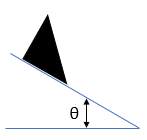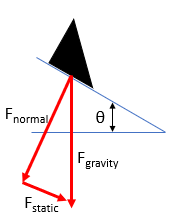## Sliding Down a Ramp

Consider a triangular block mass is resting on an adjustable ramp in the figure below. The block begins to slide when the ramp is rotated upwards until the angle is at 40°. Determine the static friction coefficient.Hint
If we break down the gravitational force into frictional and normal force components:Hint 2
The max friction force developed before the block slides is $$F_{static}\leq \mu F_{normal}$$
If we break down the gravitational force into frictional and normal force components:The max friction force developed before the block slides is $$F_{static}\leq \mu F_{normal}$$
$$F_{static}= \mu F_{normal}\rightarrow \mu=\frac{F_{static}}{F_{normal}}$$$$$\mu=tan(\theta)=tan(40)=0.84$$$
0.84